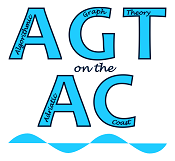#Algorithmic Graph Theory on the Adriatic Coast

Jun 16 – 19, 2015
UP FAMNIT, Koper, Slovenia
UTC timezone

## Invited Speakers

The following invited speakers have confirmed their participation:

Titles, abstracts, and slides of invited talks:

Generation of monotone graph structures
Endre Boros, Rutgers University, New Brunswick, NJ, USA
slidesMany applications require the identification of a monotone property defined on the vertices/edges of an underlying graph. Identification means the generation of all minimal (or maximal) subgraphs that satisfy the considered property (or its complement). Such applications include reliability theory (e.g., connected subgraphs), data analysis (e.g., complete bipartite subgraphs), scheduling (e.g., dominating sets), and many others. While there are strong relations between generation and counting, generating a potentially exponential family of items requires a different view of complexity, and algorithmic efficiency. In this talk I review the basic complexity notions of generation, present some of the standard algorithmic techniques, and recall some of the recent and not that recent results that illustrate these techniques.

From Matchings to Independent Sets
Vadim V. Lozin, University of Warwick, Coventry, UK
slidesIn 1965, Jack Edmonds proposed his celebrated polynomial-time algorithm to find a maximum matching in a graph. It is well known that the maximum matching problem is a special case of the more general problem of finding a maximum independent set in a graph. More precisely, the maximum matching problem is equivalent to the maximum independent set problem restricted to the class of line graphs. For general graphs, the maximum independent set problem is known to be NP-hard. What makes this problem easy in the class of line graphs and what other restrictions can lead to an efficient solution of the problem? In this talk, we analyse these and related questions.

We also review various techniques that may lead to efficient algorithms for the maximum independent set problem in restricted graph families, with a focus given to augmenting graphs and graph transformations. Both techniques have been used in the solution of Edmonds to the maximum matching problem, i.e., in the solution to the maximum independent set problem in the class of line graphs. We survey various results that exploit these techniques beyond the line graphs.  ​

Constructive algorithm for rank-width of graphs and path-width/branch-width of matroids
Sang-il Oum, KAIST, Daejeon, South Korea
slidesBranch-width and path-width are width parameters of graphs and matroids, which measure how easy it is to decompose a graph or a matroid into a tree-like or path-like structure via separations of small order. These parameters have been used not only for designing efficient algorithms with the inputs of small branch-width or path-width, but also for proving theoretical structural theorems by providing a rough structural description.

We will describe a polynomial-time algorithm to construct a path-decomposition or a branch-decomposition of width at most $k$, if it exists, for a matroid represented over a fixed finite field for fixed $k$. Our approach is based on the dynamic programming combined with the idea developed by Bodlaender for his work on tree-width of graphs. For path-width, this is a new result. For branch-width, this improves the previous work by Hlineny and Oum (Finding branch-decompositions and rank-decompositions, SIAM J. Comput., 2008) which was very indirect; their algorithm is based on the upper bound on the size of minor obstructions proved by Geelen et al. (Obstructions to branch-decompositions of matroids, JCTB, 2006) and requires testing minors for each of these obstructions. Our new algorithm does not use minor obstructions.
As a corollary, for graphs, we obtain a more direct algorithm to construct a rank-decomposition of width at most $k$ if it exists for fixed $k$.

This is joint work with Jisu Jeong (KAIST) and Eun Jung Kim (CNRS-LAMSADE).

Algorithms and Combinatorics on the Erdős–Pósa property
Dimitrios Thilikos, National and Kapodistrian University of Athens, Greece and CNRS, LIRMM, France
slidesA class of graphs C satisfies the Erdős–Pósa property for the graph class F with gap function f if, for every integer k and every graph G in F, either G contains k vertex-disjoint subgraphs, each isomorphic to a graph in C, or there is a subset S of V(G) of at most f(k) vertices (resp. edge) such that G∖S has no subgraph in C. In 1965, Erdős and Pósa proved that the set of all cycles satisfies this property for all graphs with gap O(k log k). Since then, many advances in graph theory aimed at detecting classes C and F where such a property holds and, when this is the case, optimizing the corresponding gap function. In this talk we survey some recent combinatorial and algorithmic results and open problems in this direction.